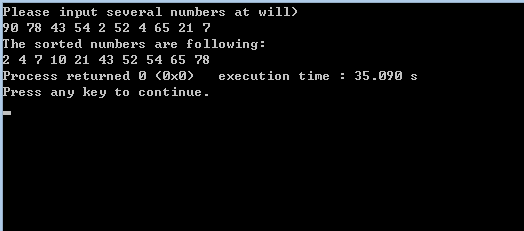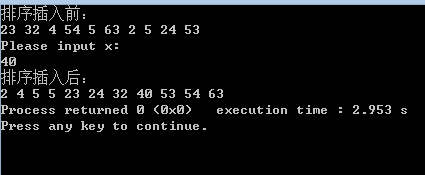• ## C++ sort排序函数用法

万次阅读 多人点赞 2017-07-27 21:35:06
最近在刷ACM经常用到排序，以前老是写冒泡，可把冒泡带到OJ里后发现经常超时，所以本想用快排，可是很多学长推荐用sort函数，因为自己写的快排写不好真的没有sort快，所以毅然决然选择sort函数 用法 1、sort函数...

最近在刷ACM经常用到排序，以前老是写冒泡，可把冒泡带到OJ里后发现经常超时，所以本想用快排，可是很多学长推荐用sort函数，因为自己写的快排写不好真的没有sort快，所以毅然决然选择sort函数

用法

1、sort函数可以三个参数也可以两个参数，必须的头文件#include < algorithm>和using namespace std; 2、它使用的排序方法是类似于快排的方法，时间复杂度为n*log2(n)

3、Sort函数有三个参数：（第三个参数可不写）

（1）第一个是要排序的数组的起始地址。

（2）第二个是结束的地址（最后一位要排序的地址）

（3）第三个参数是排序的方法，可以是从大到小也可是从小到大，还可以不写第三个参数，此时默认的排序方法是从小到大排序。

两个参数用法
#include <iostream>
#include <algorithm>
int main()
{
int a={2,4,1,23,5,76,0,43,24,65},i;
for(i=0;i<20;i++)
cout<<a[i]<<endl;
sort(a,a+20);
for(i=0;i<20;i++)
cout<<a[i]<<endl;
return 0;
}


输出结果是升序排列。（两个参数的sort默认升序排序）

三个参数
// sort algorithm example
#include <iostream>     // std::cout
#include <algorithm>    // std::sort
#include <vector>       // std::vector

bool myfunction (int i,int j) { return (i<j); }//升序排列
bool myfunction2 (int i,int j) { return (i>j); }//降序排列

struct myclass {
bool operator() (int i,int j) { return (i<j);}
} myobject;

int main () {
int myints = {32,71,12,45,26,80,53,33};
std::vector<int> myvector (myints, myints+8);               // 32 71 12 45 26 80 53 33

// using default comparison (operator <):
std::sort (myvector.begin(), myvector.begin()+4);           //(12 32 45 71)26 80 53 33

// using function as comp
std::sort (myvector.begin()+4, myvector.end(), myfunction); // 12 32 45 71(26 33 53 80)
//std::sort (myints,myints+8,myfunction);不用vector的用法

// using object as comp
std::sort (myvector.begin(), myvector.end(), myobject);     //(12 26 32 33 45 53 71 80)

// print out content:
std::cout << "myvector contains:";
for (std::vector<int>::iterator it=myvector.begin(); it!=myvector.end(); ++it)//输出
std::cout << ' ' << *it;
std::cout << '\n';

return 0;
}


string 使用反向迭代器来完成逆序排列
#include <iostream>
using namespace std;
int main()
{
string str("cvicses");
string s(str.rbegin(),str.rend());
cout << s <<endl;
return 0;
}
//输出：sescivc

展开全文C++
• package org.company.project.test; import java.util.Arrays; import java.util.Random;... //利用函数自动排序 public static void main(String[] args) { Random ra = new Random(); int[] a
package org.company.project.test;
import java.util.Arrays;
import java.util.Random;
public class ArraysSort {
//利用函数自动排序
public static void main(String[] args) {
Random ra = new Random();
int[] arr = new int;

//给数组随机赋值100以内的整数（0~99）
for(int i=0;i
arr[i] = ra.nextInt(100);
}
System.out.println("随机生成的arr：\n"+Arrays.toString(arr));

//利用函数自动排序
Arrays.sort(arr);
System.out.println("函数自动排序后的arr ：\n"+Arrays.toString(arr));

//逆序
int[] arr1 = new int[arr.length];
for(int i=0;i
arr1[arr.length-1-i] = arr[i];
}
System.out.println("逆序后的arr1 ：\n"+Arrays.toString(arr1));
}
}
运行结果：
------------------------------
随机生成的arr：
[81, 26, 96, 82, 17, 46, 88, 40, 25, 39]
函数自动排序后的arr ：
[17, 25, 26, 39, 40, 46, 81, 82, 88, 96]
逆序后的arr1 ：
[96, 88, 82, 81, 46, 40, 39, 26, 25, 17]
展开全文• //Title冒泡排序利用函数调用 //Author: //flyingostrich //Data： //Tuesday 26-August-2014 //Environment： //Codeblocks #include #define M 10//宏定义修改M的值 void Bubble_sort(int a[]) { ...
//Title冒泡排序，利用函数调用
#include<stdio.h> #define M 10//宏定义修改M的值

void Bubble_sort(int a[])
{     int i,j,t;     for(j=0;j<M;j++)       for(i=0;i<M-j;i++)         if(a[i]<a[i+1])         {             t=a[i];a[i]=a[i+1];a[i+1]=t;         } }//数组传递为地址传递，此函数结束后排序结束，无需返回值   main() {     int i,a[M];     printf("Please input several numbers at will)\n");     for(i=0;i<M;i++)       scanf("%d",&a[i]);     Bubble_sort(a);//函数调用，定义在调用之前就不需要声明     printf("The sorted numbers are following:\n");

for(i=0;i<M;i++)
printf("%d ",a[i]);

}//给定10个乱序的数，先排序后合理插入数
#include<stdio.h> #define M 11 void Bubble_sort(int a[])//冒泡排序 {    int i,j,temp;    for(i=0;i<9;i++)      for(j=0;j<9-i;j++)      if(a[j]>a[j+1])      {         temp=a[j];         a[j]=a[j+1];         a[j+1]=temp;      } }   void insert(int a[],int x)//插入函数 {     int i,j,temp;     for(i=0;i<10;i++)       if(x<a[i])       {           for(j=9;j>=i;j--)             a[j+1]=a[j];           break;       }     a[i]=x; }   int main() {     int x;     int a[M]={23,32,4,54,5,63,2,5,24,53};     printf("排序插入前：\n");      for(int i=0;i<M-1;i++)       printf("%d ",a[i]);     printf("\n");     Bubble_sort(a);     printf("Please input x:\n");     scanf("%d",&x);     insert(a,x);     printf("排序插入后：\n");     for(int i=0;i<M;i++)       printf("%d ",a[i]);     return 0; }

结果如下：展开全文c语言 插入
• ## sort()函数排序vector

千次阅读 2019-05-05 14:33:26
sort()函数默认的排序方式为升序，如果需要降序排序，可以自定义排序函数 bool comp(int x ,int y) { return x > y; } 对vector进行排序时 sort(vec.begin(),vec.end(),comp); 这样排序出的vector就是降序...
sort()函数默认的排序方式为升序，如果需要降序排序，可以自定义排序函数
bool comp(int x ,int y)
{
return x > y;
}
对vector 进行排序时
sort(vec.begin(),vec.end(),comp);
这样排序出的vector就是降序
展开全文•excel c
• ## 排序函数

千次阅读 2019-01-27 17:10:43
sort()函数是对给定区间的元素进行排序，但是会改变值相同的元素的相对位置。stable_sort()是对给定区间的元素进行稳定排序，如果两个元素相等，那么排序完成后两个元素的相对位置保持不变，partial_sort()是对给定...sort
• qsort函数排序字符串数组 描述： qsort()函数是一个库函数，也是一个万能的排序函数，只需要自己写好compare()函数即可，那么怎么用qsort()函数来排序字符串呢？ 思路： 个人感觉，这个问题很好解决，困难的就是在...
• ## sort函数排序用法

千次阅读 2019-06-07 15:14:28
具体用法在上一篇博客中已经说明了，主要是sort函数实现了将数组数按从大到小的顺序给进行了排列，要注意的一点就是在头文件中声明#include跟#include，其次就是在调用函数时可以是sort（a.begin(),a.end()）,也可以...
• ## sort排序函数

千次阅读 2019-06-03 22:37:27
sort函数可以排序任何类型的函数，包括自己写的结构体 （sort函数效率高） 头文件 #include<algorithm> 排序数值 从小到大 int num={1,9,3,5,6,7,4,3,32,2}; //sort(num+i,num+j),从num[i]到num[j-1]...sort
• 划重点：添加cmp（函数名可更改）函数，根据需要定制排序 #include &lt;cstdio&gt; #include &lt;algorithm&gt; using namespace std; struct Node { int a; int b; }; int cmp(const Node &...
• ## sort函数自定义排序

千次阅读 2020-12-15 18:09:29
sort函数自定义排序 咳咳，自定义排序说实话用的地方还是很多的，像ACM里面，天梯赛里面，特别是天梯，必出这玩意。。。咳咳，水点字数，咱继续。 首先哈，我们来看一下c++自带的排序； 可以看出哈，默认是按...c++
• //冒泡排序的两种形式 for(i=0;i;i++) { for(j=i;j;j++) { if(p(a[i],a[j])>0) { int temp; temp = a[j]; a[j] = a[i]; a[i]=temp; } } } } void print(int *a,int n) { int...
• 利用冒泡排序、直接选择排序分别实现指定数组的升序、降序排列，并可以选择指定排序函数。 Python代码如下： #冒泡排序法实现升序排列 def bubble_sort(lists, sort = None): if not sort: count = len(lists) ...python bubble
• 使用sort()函数进行排序会调用toString()函数，然后比较字符的ASCII码进行排序。但是sort()函数可以接受一个函数，通过函数的返回值进行控制排序元素的位置。 function compare(value1, value2) { if (value1 <...
• #include int compare(int a,int b) { return a - b; } void select_sort(int *a,int n,int(*p)(int,int)) { int i,j; for(i=0;i;i++) { for(j=i+1;j;j++) { if(p(a[i],a[j])>0) ... int tem
• /*外循环控制排序趟数，n个数排n-1趟*/ for (i= 0 ;i< 9 -j;i++) /*内循环每趟比较的次数，第j趟比较n-j次*/ if (a[i]>a[i+ 1 ]) /*相邻元素比较，逆序则交换*/ { t=a[i]; a[i]=a[i+ 1 ]; a[i+ 1 ]=t; ...
• ## Mysql排序函数

千次阅读 2018-04-19 17:16:40
一、row_numberrow_number会为查询出来的每条记录生成一个序号，依次排序并且不会重复，row_number必须要使用over句子选择对某一列进行排序才会生成序号，row_number用法实例：select ROW_NUMBER() OVER(order by ...Mysql
• sort()函数默认是升序排序，只能应用于C#指定数据类型，但这里要和大家分享的是自定义Sort函数排序的对象和规则，方便大家去选择适合自己项目的排序。 代码实现： using System; using System.Collections; using...Sort
• Python2中的**自定义布尔函数cmp=custom_cmp(x, y)**由Python3中的**key=custom_key(x)**代替。...在python3中，待比较元素x通过custom_key函数转化为Python能比较的值custom_key(x)，进而再基于返回值进行排序python
• ## 冒泡排序法函数

千次阅读 2017-10-04 23:58:49
冒泡排序法的函数实现 使用教程冒泡排序法的函数实现话不多说上代码，拿去直接用。// 冒泡排序函数 /* * @brief sort * @param array为数组名称，length为数组的长度，order为1或0,1代表从小到大排序 * 0代表从大到...c语言
• 利用 Array.Sort()函数对数组排序，c#写的简单控制台应用程序
• 最近有个需求是对sql的结果集list进行排序，因为list默认查出的结果排序是按该表id...2，在网上看到 instr 的函数来进行排序，这里我想要说的是在hql中拼接该语句时候赢注意的问题  先看一下 例句：  SELECT * FInstr instr list List LIST
• 关于南大通运8ampp数据库的排序函数介绍 RANK OVER函数 RANK() OVER([PARTITION BY col_name1,col_name2,…] ORDER BY col_name1 [ASC/DESC], col_name2 [ASC/DESC],…) 功能描述： 根据 ORDER BY 子句中表达式...数据库 南大通用
• ## 数组排序函数

千次阅读 2018-07-07 10:06:15
sort() 函数用于对数组单元从低到高进行排序。 　rsort() 函数用于对数组单元从高到低进行排序。 　asort() 函数用于对数组单元从低到高进行排序并保持索引关系。 　arsort() 函数用于对数组单元从高到低进行排序...
• ## Python排序函数

千次阅读 2019-07-26 11:48:05
Python中自带了两个排序函数sort和sorted，用法分别为： sort函数用法 list.sort(cmp=None, key=None, reverse=False) 特点：对list原地排序（直接改变数组），无返回值。 参数： cmp——可选参数， 可用于...
• 功 能：使用快速排序例程进行排序   用 法：void qsort(void *base,int n...参数：base是排序的一个集合数组，num是这个数组元素的个数，width是一个元素的大小，comp是一个比较函数   比如：    ① 对一个长为100C语言
• ## Python3 list 排序函数详解

万次阅读 多人点赞 2018-05-28 18:55:03
Python3 list 排序函数详解 一、列表的sort排序函数 函数原型： list.sort(key=None,reverse=False) 函数功能： 对原列表进行排序，完成排序后，原列表变为有序列表。默认情况（不传入任何参数时）按字典顺序...list sort
• 这篇文章主要介绍了Python匿名函数/排序函数/过滤函数/映射函数/递归/二分法 ,本文通过实例代码给大家介绍的非常详细，具有一定的参考借鉴价值，需要的朋友可以参考下 一. lamda匿名函数 　为了解决一些简单的需求而...python 大数据
• c++有自带的sort排序函数，比自己写的冒泡算法快很多，可以拿来直接用 1、加上头文件#include &lt;algorithm&gt; ,using namespace std 2、函数使用方法：sort(首元素地址，尾元素地址的下一个地址，比较......